Home > CCA2 > Chapter 8 > Lesson 8.3.3 > Problem8-177

8-177.
1. Graph two cycles of each function. Homework Help ✎

1. y = −2cos(x +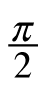)

2. y = sin(x)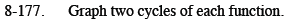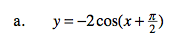What is the amplitude?Where is the locator point? What is the orientation? What is the period?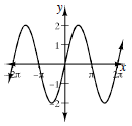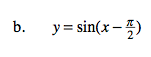See part (a).